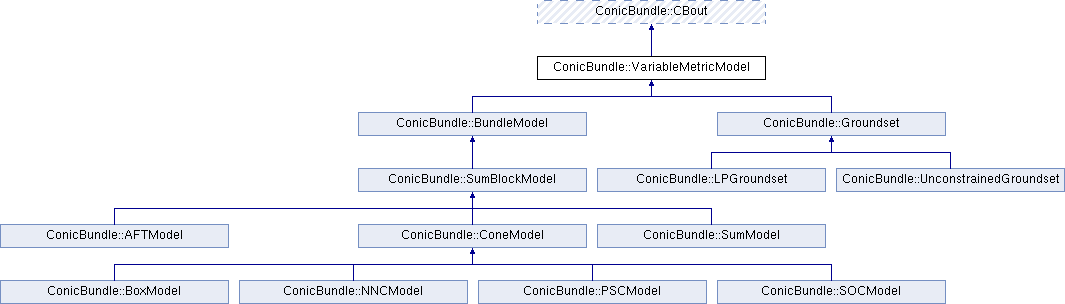ConicBundle
ConicBundle::VariableMetricModel Class Reference

declares the interface that a BundelModel needs to provide for contributing to VariableMetric information More...

`#include <VariableMetric.hxx>`

Inheritance diagram for ConicBundle::VariableMetricModel:## Public Member Functions

VariableMetricModel (CBout *cb=0, int cbincr=-1)
constructor for passing on ouptut information

virtual ~VariableMetricModel ()
virtual destructor

virtual int add_variable_metric (VariableMetric &H, CH_Matrix_Classes::Integer y_id, const CH_Matrix_Classes::Matrix &y, bool descent_step, CH_Matrix_Classes::Real weightu, CH_Matrix_Classes::Real model_maxviol, const CH_Matrix_Classes::Indexmatrix *indices=0)
add to the variable metric information H some model dependent "second order" information of the function modelled More...

virtual VariableMetricModelvariable_metric_transform ()
Overload this in order apply transformations in between. More...Public Member Functions inherited from ConicBundle::CBout
virtual void set_out (std::ostream *out=0, int print_level=1)
Specifies the output level (out==NULL: no output at all, out!=NULL and level=0: errors and warnings, level>0 increasingly detailed information) More...

virtual void set_cbout (const CBout *cb, int incr=-1)
Specifies the output level relative to the given CBout class. More...

void clear_cbout ()
reset to default settings (out=0,print_level=1)

CBout (const CBout *cb=0, int incr=-1)
calls set_cbout

CBout (std::ostream *outp, int pl=1)
initialize correspondingly

CBout (const CBout &cb, int incr=0)
copy constructor

virtual bool cb_out (int pl=-1) const
Returns true if out!=0 and (pl<print_level), pl<0 should be used for WARNINGS and ERRORS only, pl==0 for usual output.

std::ostream & get_out () const
If cb_out() returned true, this returns the output stream, but it will abort if called with out==0.

std::ostream * get_out_ptr () const
returns the pointer to the output stream

int get_print_level () const
returns the print_level

virtual int mfile_data (std::ostream &out) const
writes problem data to the given outstream

## Detailed Description

declares the interface that a BundelModel needs to provide for contributing to VariableMetric information

## Member Function Documentation

 virtual int ConicBundle::VariableMetricModel::add_variable_metric ( VariableMetric & H, CH_Matrix_Classes::Integer y_id, const CH_Matrix_Classes::Matrix & y, bool descent_step, CH_Matrix_Classes::Real weightu, CH_Matrix_Classes::Real model_maxviol, const CH_Matrix_Classes::Indexmatrix * indices = `0` )
virtual

add to the variable metric information H some model dependent "second order" information of the function modelled

The routine may add nothing if there is not sufficient information. Otherwise see the functions in VariableMetric for how to enter information depending on how H supports dynamic scaling.

Parameters
 [in,out] H BundleScaling H holds the current scaling matrix, provides the information needed to add to it. [in] y_id the point id of the point supplied [in] y the scaling should be relevant around this point, e.g. in the smooth case the (nonnegative) diagonal or low rank representation of its Hessian [in] descent_step true if and only if the solver just performed a descent step; if not true, the dynamic scaling routine may only add further semidefintie matrices but may not make the term smaller in the semidefinite sense. [in] weightu the positive scalar value currently used for weighting the quadratic (trust region) term [in] model_maxviol for nonsmooth functions the aggregate together with the quadratic term should aim at keeping the value above the value of the minorant minus this model_maxviol (where all minorants are assumed or shifted to cut below the aggregate by at least .1 this value) [in] indices if not NULL, the effect of the changes of the quadratic term must be restricted to these indices
Returns
• 0 ... on success or if not supported (= adds nothing),
• 1 ... on failure,

Reimplemented in ConicBundle::SumModel, ConicBundle::SumBlockModel, ConicBundle::LPGroundset, and ConicBundle::AFTModel.

## ◆ variable_metric_transform()

 virtual VariableMetricModel* ConicBundle::VariableMetricModel::variable_metric_transform ( )
inlinevirtual

Overload this in order apply transformations in between.

VariableMetric never calls the above routines directly but always via a call to transform. This allows to insert affine function transformations, if so desired.

Reimplemented in ConicBundle::BundleModel.

The documentation for this class was generated from the following file: